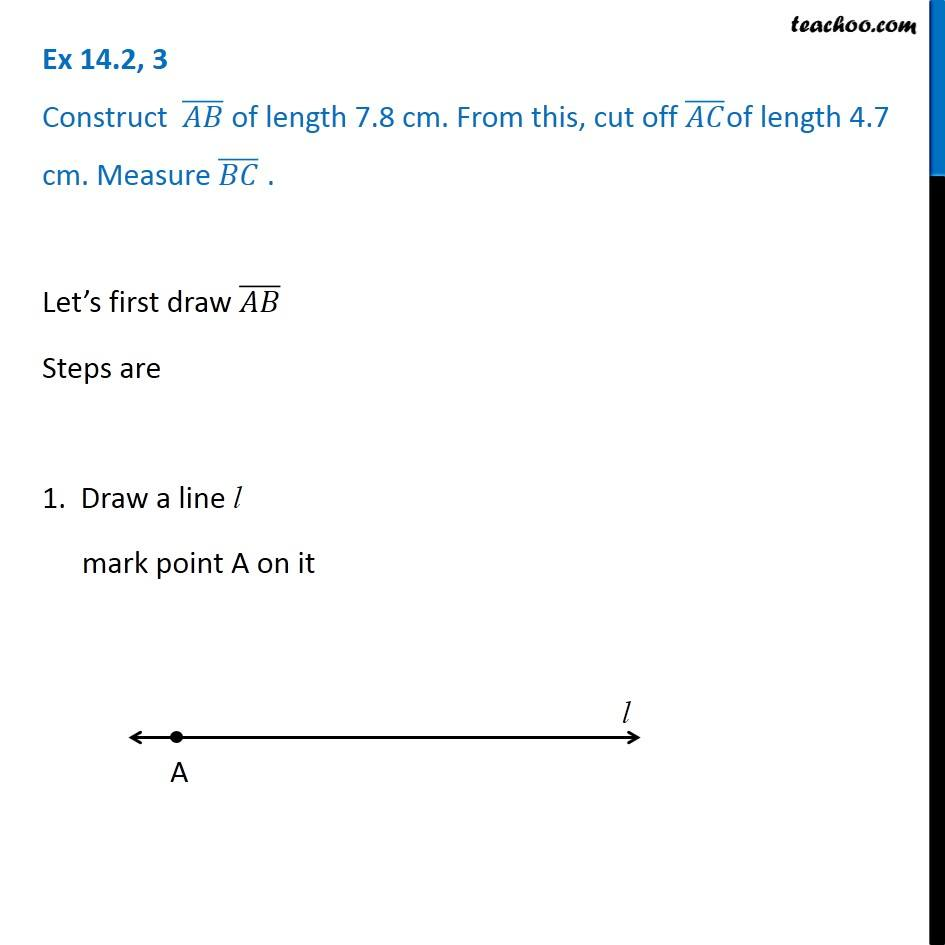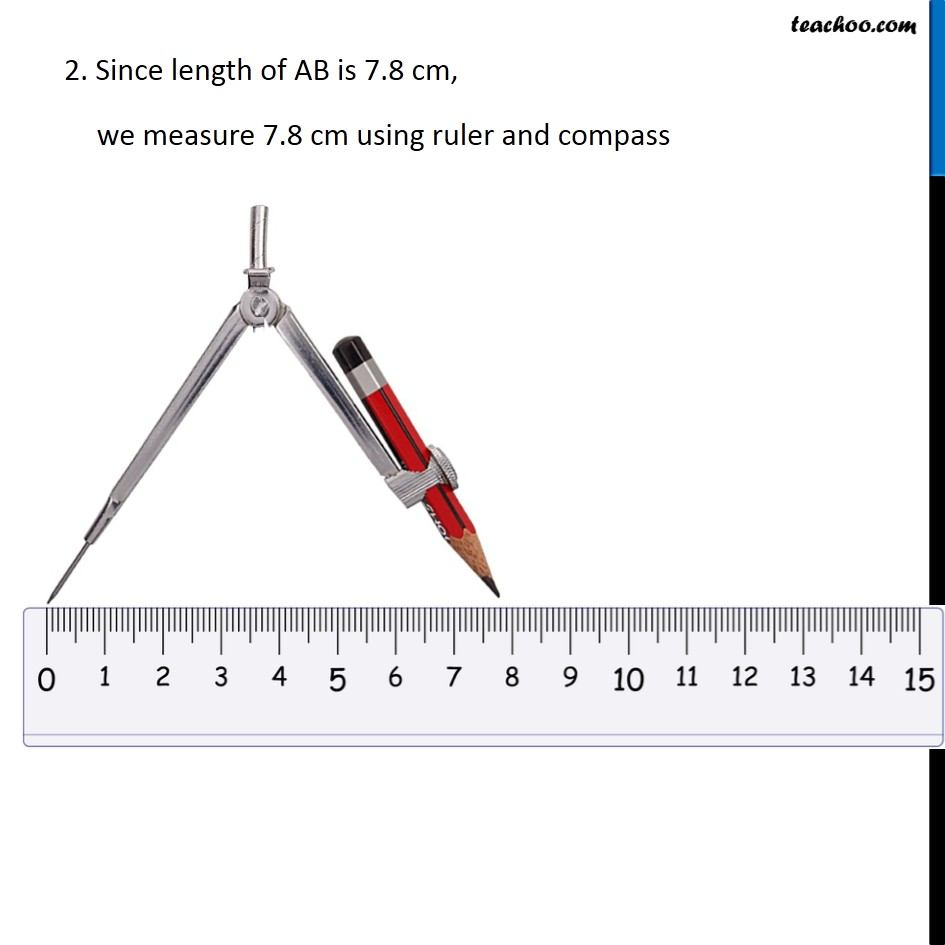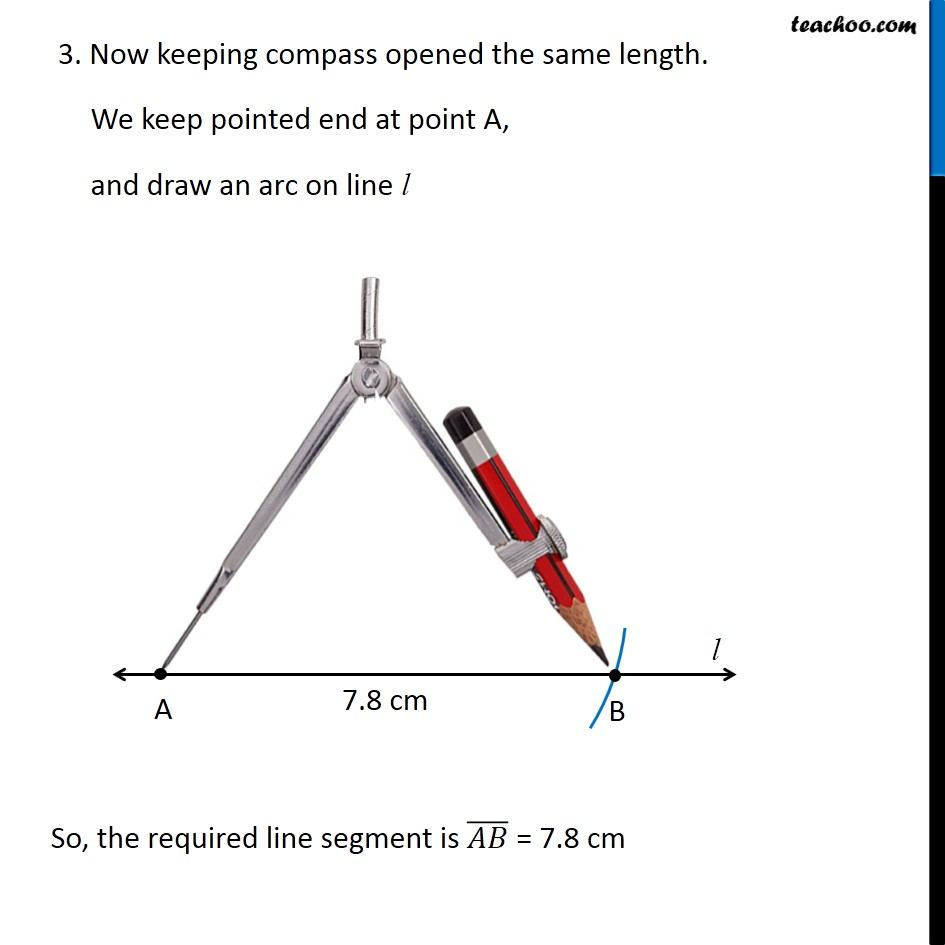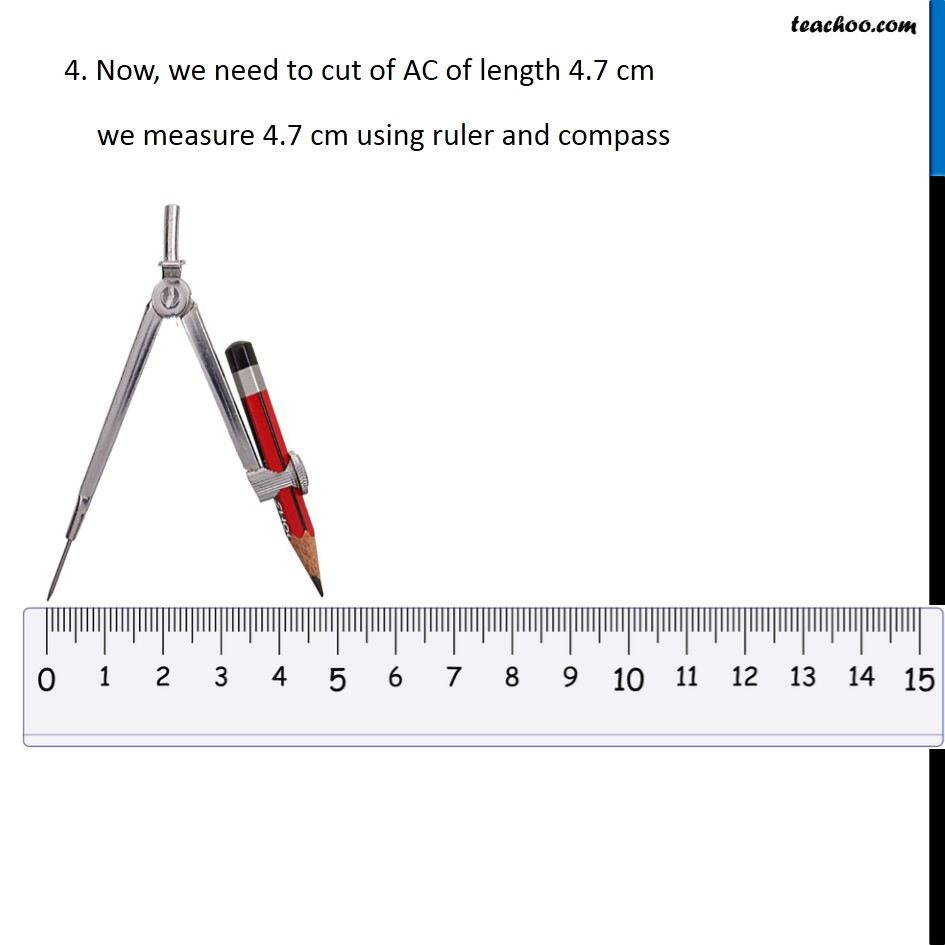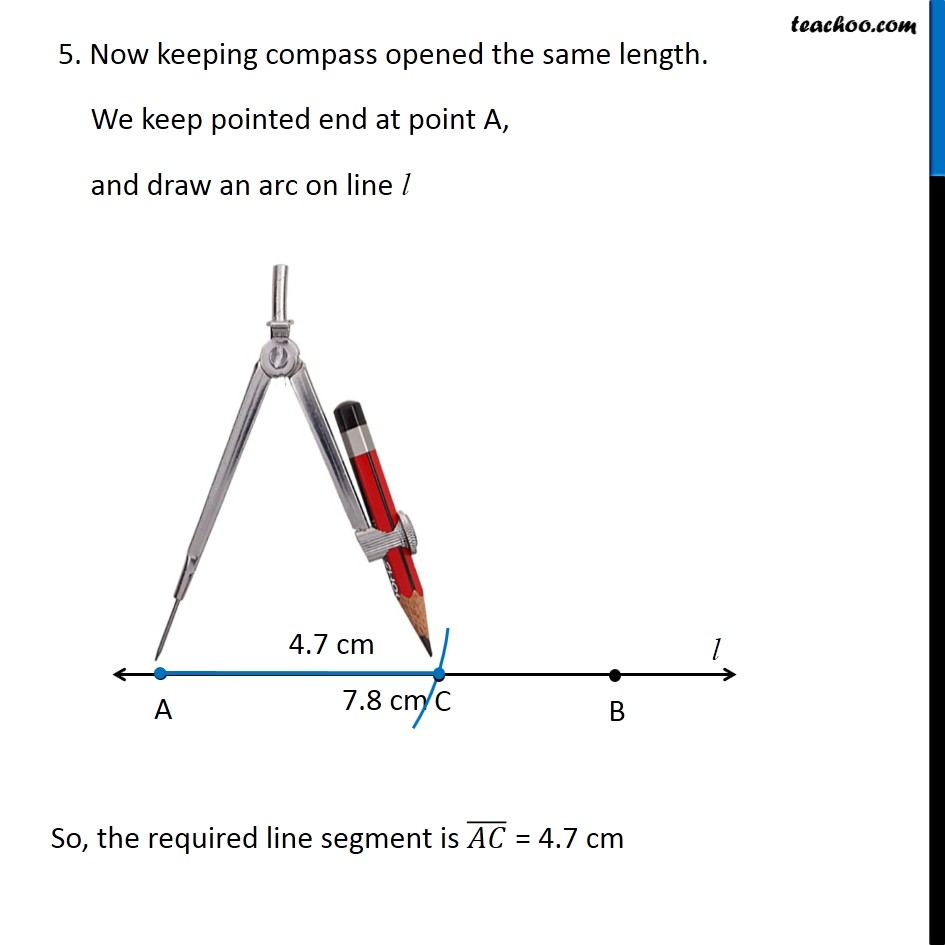Subscribe to our Youtube Channel - https://you.tube/teachoo

1. Chapter 14 Class 6 Practical Geometry
2. Concept wise
3. Construction of a line segment of a given length

Transcript

Ex 14.2, 3 Construct (𝐴𝐵) ̅ of length 7.8 cm. From this, cut off (𝐴𝐶) ̅of length 4.7 cm. Measure (𝐵𝐶) ̅ . Let’s first draw (𝐴𝐵) ̅ Steps are 1. Draw a line l mark point A on it 2. Since length of AB is 7.8 cm, we measure 7.8 cm using ruler and compass 3. Now keeping compass opened the same length. We keep pointed end at point A, and draw an arc on line l So, the required line segment is (𝐴𝐵) ̅ = 7.8 cm So, the required line segment is (𝐴𝐵) ̅ = 7.8 cm 4. Now, we need to cut of AC of length 4.7 cm we measure 4.7 cm using ruler and compass 5. Now keeping compass opened the same length. We keep pointed end at point A, and draw an arc on line l So, the required line segment is (𝐴𝐶) ̅ = 4.7 cm

Construction of a line segment of a given length

About the AuthorDavneet Singh
Davneet Singh is a graduate from Indian Institute of Technology, Kanpur. He has been teaching from the past 9 years. He provides courses for Maths and Science at Teachoo.